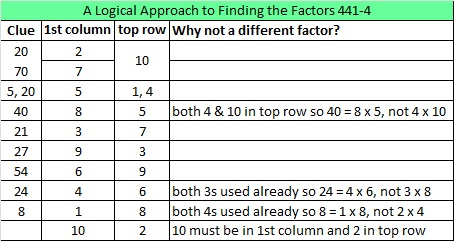# 264 and Level 4

• 264 is a composite number.
• Prime factorization: 264 = 2 x 2 x 2 x 3 x 11, which can be written (2^3) x 3 x 11
• The exponents in the prime factorization are 3, 1, and 1. Adding one to each and multiplying we get (3 + 1)(1 + 1)(1 + 1) = 4 x 2 x 2 = 16. Therefore 264 has 16 factors.
• Factors of 264: 1, 2, 3, 4, 6, 8, 11, 12, 22, 24, 33, 44, 66, 88, 132, 264
• Factor pairs: 264 = 1 x 264, 2 x 132, 3 x 88, 4 x 66, 6 x 44, 8 x 33, 11 x 24, or 12 x 22
• Taking the factor pair with the largest square number factor, we get √264 = (√4)(√66) = 2√66 ≈ 16.248That’s a lot of factors. Finding the factors for this puzzle will be much easier:Print the puzzles or type the factors on this excel file: 10 Factors 2014-10-13